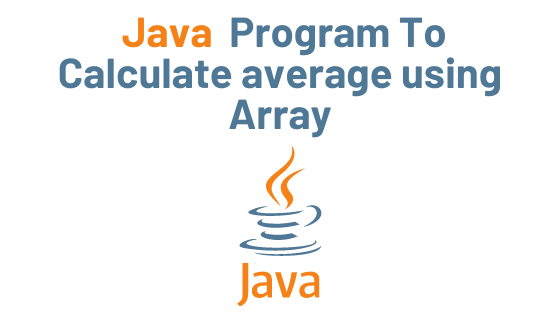## Java Program to Calculate average using Array with Example

We’ll look at two programs that use arrays to find the average of numbers. First, the program computes the average of the specified array elements. The second program takes the value of n (the number of elements) and the numbers supplied by the user and computes the average of them using an array.

Example : Program to find the average of numbers using an array

```public class JavaExample {

public static void main(String[] args) {
double[] arr = {19, 12.89, 16.5, 200, 13.7};
double total = 0;

for(int i=0; i<arr.length; i++){
total = total + arr[i];
}

/* arr.length returns the number of elements
* present in the array
*/
double average = total / arr.length;

/* This is used for displaying the formatted output
* if you give %.4f then the output would have 4 digits
* after decimal point.
*/
System.out.format("The average is: %.3f", average);
}
}```

Output:

The average is: 52.418

Example 2: Calculate the average of numbers entered by the user

In this example, we’re going to use Scanner to get the value of n as well as all of the numbers from the user.

```public class JavaExample {

public static void main(String[] args) {
System.out.println("How many numbers you want to enter?");
Scanner scanner = new Scanner(System.in);
int n = scanner.nextInt();
/* Declaring array of n elements, the value
* of n is provided by the user
*/
double[] arr = new double[n];
double total = 0;

for(int i=0; i<arr.length; i++){
System.out.print("Enter Element No."+(i+1)+": ");
arr[i] = scanner.nextDouble();
}
scanner.close();
for(int i=0; i<arr.length; i++){
total = total + arr[i];
}

double average = total / arr.length;

System.out.format("The average is: %.3f", average);
}
}```

Output:

How many numbers you want to enter?
5
Enter Element No.1: 12.7
Enter Element No.2: 18.9
Enter Element No.3: 20
Enter Element No.4: 13.923
Enter Element No.5: 15.6
The average is: 16.225

theudaipurstore.com

Calculate average using Array Calculate average using Array Calculate average using Array Calculate average using Array Calculate average using Array Calculate average using Array Calculate average using Array Calculate average using Array In Java, encapsulation is a mechanism for combining variables (data) and methods (code) into a single unit. It is the process of concealing information details and safeguarding the object’s data and behavior. It’s one of the four key concepts in OOP. Because the encapsulating class is simple to test, it’s ideal for unit testing.In Java, encapsulation is a mechanism for combining variables (data) and methods (code) into a single unit. It is the process of concealing information details and safeguarding the object’s data and behavior. It’s one of the four key concepts in OOP. Because the encapsulating class is simple to test, it’s ideal for unit testing.In Java, encapsulation is a mechanism for combining variables (data) and methods (code) into a single unit. It is the process of concealing information details and safeguarding the object’s data and behavior. It’s one of the four key concepts in OOP. Because the encapsulating class is simple to test, it’s ideal for unit testing.In Java, encapsulation is a mechanism for combining variables (data) and methods (code) into a single unit. It is the process of concealing information details and safeguarding the object’s data and behavior. It’s one of the four key concepts in OOP. Because the encapsulating class is simple to test, it’s ideal for unit testing.In Java, encapsulation is a mechanism for combining variables (data) and methods (code) into a single unit. It is the process of concealing information details and safeguarding the object’s data and behavior. It’s one of the four key concepts in OOP. Because the encapsulating class is simple to test, it’s ideal for unit testing.In Java, encapsulation is a mechanism for combining variables (data) and methods (code) into a single unit. It is the process of concealing information details and safeguarding the object’s data and behavior. It’s one of the four key concepts in OOP. Because the encapsulating class is simple to test, it’s ideal for unit testing.In Java, encapsulation is a mechanism for combining variables (data) and methods (code) into a single unit. It is the process of concealing information details and safeguarding the object’s data and behavior. It’s one of the four key concepts in OOP. Because the encapsulating class is simple to test, it’s ideal for unit testing.In Java, encapsulation is a mechanism for combining variables (data) and methods (code) into a single unit. It is the process of concealing information details and safeguarding the object’s data and behavior. It’s one of the four key concepts in OOP. Because the encapsulating class is simple to test, it’s ideal for unit testing.In Java, encapsulation is a mechanism for combining variables (data) and methods (code) into a single unit. It is the process of concealing information details and safeguarding the object’s data and behavior. It’s one of the four key concepts in OOP. Because the encapsulating class is simple to test, it’s ideal for unit testing.